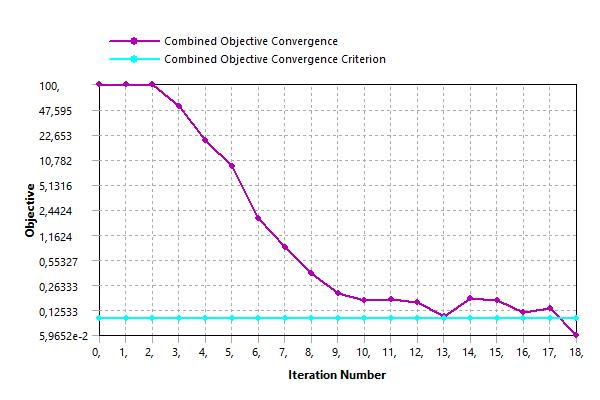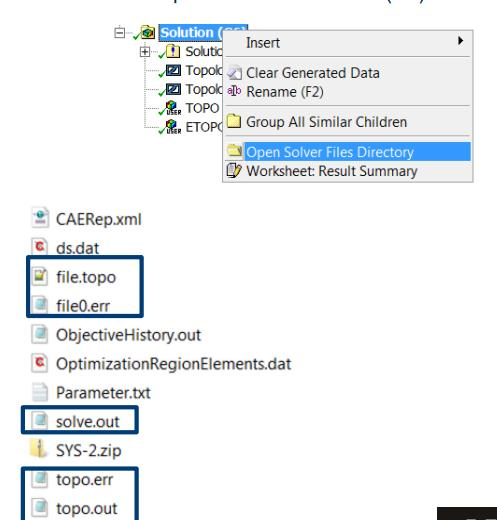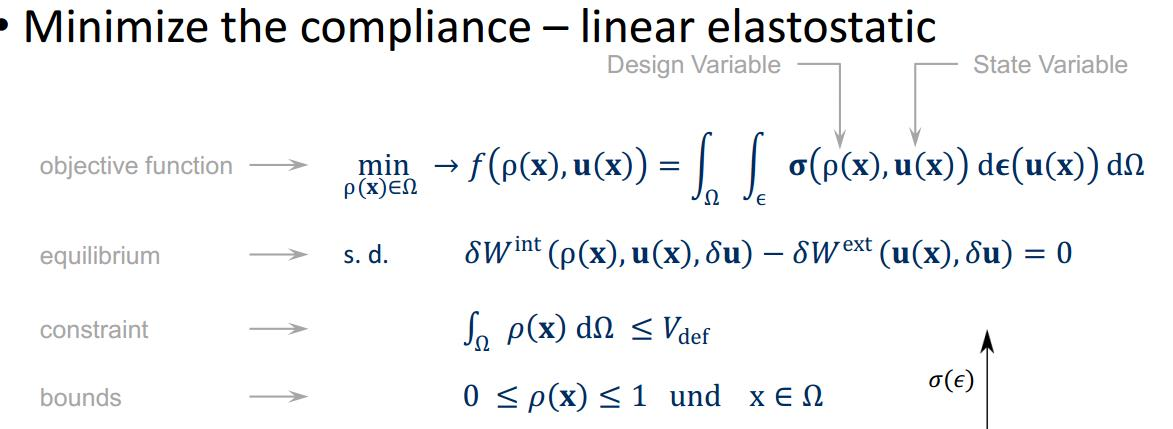# Topology optimization solution worksheet

Hello,

I have runed an topology optimization of a simple block. The Mass retain is 3 %. In this picture the solution information worksheet is presented. My first question is, is there any option to export these data to an excel sheet?

Question to the picture: Which unit is the y-axis (Objectiv)? And Where do the values come from? I don't understand how to interpret this axis.I know this axis runs to zero to minimize the Compliance which stands for maximization of the stiffness. Maybe you can help me to find out how to present my results correctly. Thank you!• You probably can't export these values but if you right-click on the solution and open the solver files directory, you should see the following files:The values, displayed here are an echo of what is written to these files. Check the Objective History as well.

The objective function is nothing but your strain energy density when trying to minimize structural compliance. It is recommended to use your mass as a constraint.You can find more details on the methodology in the help and the subsequent references provided.

• edited November 2019

Thank you very much!

So the objective function calculates the stress (o), which depends on the density (p) and the shift (u), wants to minimize this function.

Does the function calculate every node? I have problems to understand how the values on the y-axis are calculate exactly.

• (p) is the pseudo-density a value between 0 and 1 and u is the displacement of your structure. The objective function is nothing but your strain energy density. The value on the y-axis is a normalized value of the strain energy density. By introducing and varying the pseudo densities you are trying to minimize the objective function and at the same time trying to satisfy the equilibrium function (in other words the governing equation of FEA).